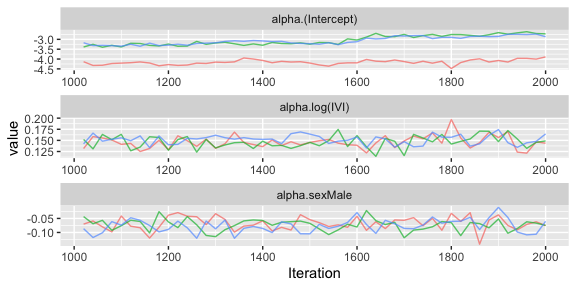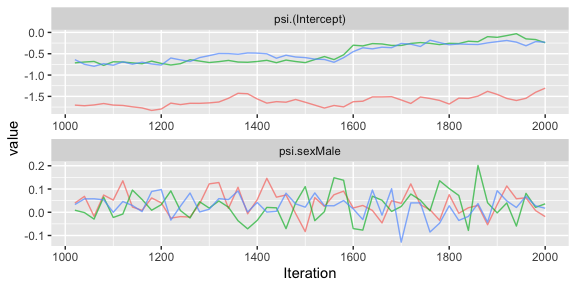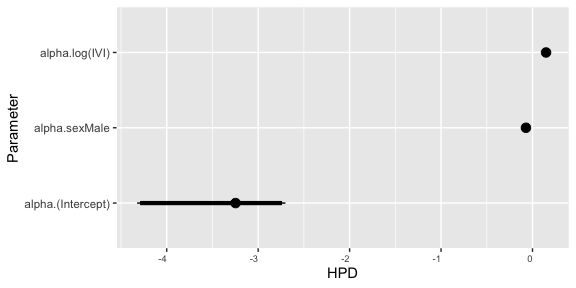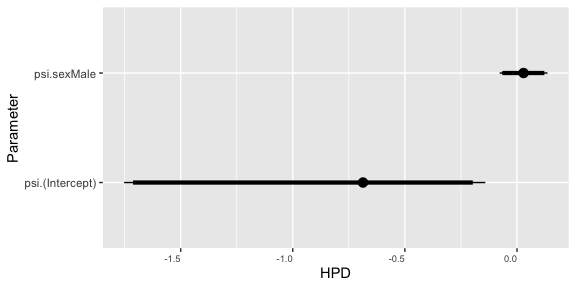# Pied Flycatchers 3: Joint Effects

#### 2021-11-22

Joint effects are fixed or random effects that take the same value in linear predictors describing variation in both the mean and the dispersion parameter. As an example, we consider the simplest model of the pied flycatcher data with only fixed effects on the mean and dispersion, except that we assume that the effect of broodsize is the same in both linear predictors.

# Library

First you have to load the package:

## Load package
library(dalmatian)

# Data

## Load pied flycatcher data
data(pied_flycatchers_1)

## Create variables bounding the true load
pfdata$lower=ifelse(pfdata$load==0,log(.001),log(pfdata$load-.049)) ## Warning in log(pfdata$load - 0.049): NaNs produced
pfdata$upper=log(pfdata$load+.05)

# Defining the Model

Our model will assume that the logarithm of the load returned on the $$j$$th trip by adult $$i$$ is normally distributed with mean $$\mu_{ij}$$ and variance $$\phi$$ where: $\mu=\alpha_0 + \alpha_1 \mathrm{log(IVI)}_{ij} + \beta_2 \mathrm{sex}_i + \gamma_1 \mathrm{broodsize}_i$ and $$$\log(\phi)=\psi_0 + \psi_1\mathrm{sex}_i$$ + _1 _i$. Here $$\gamma_1$$ is a fixed effect that is common to both the linear predictors describing the variation in both the mean and dispersion. This model can be defined with the following three components:

## Mean model
mymean <- list(fixed=list(name="alpha",
formula=~ log(IVI) + sex,
priors=list(c("dnorm",0,.001))))

## Dispersion model
mydisp=list(fixed=list(name="psi",
formula=~sex,
priors=list(c("dnorm",0,.001))),

## Joint components
myjoint <- list(fixed = list(name = "gamma",
formula = ~-1 + broodsize,
priors = list(c("dnorm",0,.001))))

These three objects will now be used to generate the JAGS code, data, and initial values for running the model. Note that a link function cannot be specified as part of the joint component.

# Running the Model

The model can then be fit with dalmatian by defining the arguments that control the construction and updating of the JAGS model. Full details are included in vignette(pied-flycatchers-1).

## Set working directory
## By default uses a system temp directory. You probably want to change this.
workingDir <- tempdir()

## Define list of arguments for jags.model()

## Define list of arguments for coda.samples()
cs.args <- list(n.iter=5000,thin=20)

## Run the model using dalmatian
pfmcmc4 <- dalmatian(df=pfdata,
mean.model=mymean,
dispersion.model=mydisp,
joint.model=myjoint,
jags.model.args=jm.args,
coda.samples.args=cs.args,
rounding=TRUE,
lower="lower",
upper="upper",
residuals = FALSE,
debug=FALSE)

# Results

The following illustrates the functions for processing the results. Again, these are fully described in vignette(pied-flycatchers-1).

## Numerical Convergence Diagnostics

## Compute convergence diagnostics
pfconvergence4 <- convergence(pfmcmc4)
## Gelman-Rubin diagnostics
pfconvergence4$gelman ## Potential scale reduction factors: ## ## Point est. Upper C.I. ## alpha.(Intercept) 4.95 10.54 ## alpha.log(IVI) 1.04 1.15 ## alpha.sexMale 1.02 1.08 ## psi.(Intercept) 5.18 11.02 ## psi.sexMale 1.01 1.03 ## gamma.broodsizeDecreased 5.03 11.18 ## gamma.broodsizeIncreased 5.07 11.35 ## Raftery diagnostics pfconvergence4$raftery
##
## Quantile (q) = 0.025
## Accuracy (r) = +/- 0.005
## Probability (s) = 0.95
##
## You need a sample size of at least 3746 with these values of q, r and s
## Effective sample size
pfconvergence4$effectiveSize ## alpha.(Intercept) alpha.log(IVI) alpha.sexMale ## 20.614124 150.000000 173.961120 ## psi.(Intercept) psi.sexMale gamma.broodsizeDecreased ## 11.452691 200.552888 8.471997 ## gamma.broodsizeIncreased ## 9.579250 ## Graphical Convergence Diagnostics ## Generate traceplots pftraceplots4 <- traceplots(pfmcmc4,show=FALSE,nthin=10) ## Fixed effects for mean pftraceplots4$meanFixed## Fixed effects for dispersion
pftraceplots4$dispersionFixed## Numerical Summaries ## Compute numerical summaries pfsummary4 <- summary(pfmcmc4) ## Print numerical summaries pfsummary4 ## ## Iterations = 1020:2000 ## Thinning interval = 20 ## Number of chains = 3 ## Sample size per chain = 50 ## ## Posterior Summary Statistics for Each Model Component ## ## Mean Model: Fixed Effects ## Mean Median SD Lower 95% Lower 50% Upper 50% Upper 95% ## (Intercept) -3.42 -3.25 0.56 -4.33 -3.35 -2.83 -2.70 ## log(IVI) 0.15 0.15 0.01 0.12 0.14 0.16 0.17 ## sexMale -0.07 -0.07 0.02 -0.12 -0.08 -0.06 -0.03 ## ## Dispersion Model: Fixed Effects ## Mean Median SD Lower 95% Lower 50% Upper 50% Upper 95% ## (Intercept) -0.86 -0.69 0.56 -1.77 -0.71 -0.23 -0.17 ## sexMale 0.03 0.03 0.05 -0.09 0.00 0.06 0.13 ## ## Joint Model: Fixed Effects ## Mean Median SD Lower 95% Lower 50% Upper 50% Upper 95% ## broodsizeDecreased 0.13 -0.03 0.56 -0.56 -0.47 0.03 1.05 ## broodsizeIncreased 0.22 0.06 0.56 -0.53 -0.36 0.12 1.08 ## Graphical Summaries ## Generate caterpillar pfcaterpillar4 <- caterpillar(pfmcmc4,show = FALSE) ## Fixed effects for mean pfcaterpillar4$meanFixed## Fixed effects for dispersion
pfcaterpillar4\$dispersionFixed